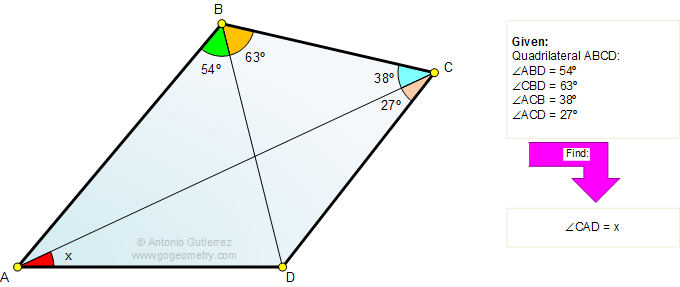Problem 695: Triangle, Angles, Auxiliary Construction, Congruence, Mind Map, Polya. Level: High School, College, Math Ed. The figure shows a quadrilateral ABCD with angle ABD = 54 degrees, angle DBC = 63 degrees, angle ACB = 38 degrees, and angle ACD = 27 degrees, Find the measure of the angle CAD.How to Solve It, Interactive Mind Map George Pólya's 1945 book "How to Solve It, A new aspect of Mathematical Method", is a book describing methods of problem solving. It suggests the following steps when solving a mathematical problem: (1) First, you have to understand the problem. (2) After understanding, then make a plan. (3) Carry out the plan. (4) Look back on your work. How could it be better?    Mind Map Help. To see a note: Hover over a yellow note button. To Fold/Unfold: click a branch. To Pan: click and drag the map canvas.Recent Additions